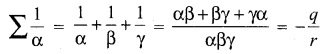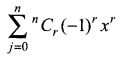## Tamilnadu Samacheer Kalvi 12th Maths Solutions Chapter 3 Theory of Equations Ex 3.7

Choose the correct or the most suitable answer from the given four alternatives:

Question 1.
A zero of x3 + 64 is _______
(a) 0
(b) 4
(c) 4i
(d) -4
(d) -4
Hint: x3 + 64 = 0
⇒ x3 = -64
⇒ x3 = (-4)3
⇒ x = -4

Question 2.
If f and g are polynomials of degrees m and n respectively, and if h(x) = (f 0 g) (x), then the degree of h is ______
(a) mn
(b) m + n
(c) mn
(d) nm
(a) mnQuestion 3.
A polynomial equation in x of degree n always has _______
(a) n distinct roots
(b) n real roots
(c) n imaginary roots
(d) at most one root.
(c) n imaginary roots (Every real number is also imaginary)

Question 4.
If α, β and γ are the zeros of x3 + px2 + qx + r, then $$\sum \frac{1}{\alpha}$$ is ______
(a) $$-\frac{q}{r}$$
(b) $$\frac{q}{p}$$
(c) $$\frac{q}{r}$$
(d) $$-\frac{q}{p}$$
(a) $$-\frac{q}{r}$$
Hint:Question 5.
According to the rational root theorem, which number is not possible rational zero of 4x7 + 2x4 – 10x3 – 5?
(a) -1
(b) $$\frac{5}{4}$$
(c) $$\frac{4}{5}$$
(d) 5
(c) $$\frac{4}{5}$$
Hint:
an = 4; a0 = 5
Let $$\frac{p}{q}$$ be the root of P (x). P must divide 5, possible values of P are ±1, ±5
q must divide 4, possible values of q are ±1, ±2, ±4
Possible roots are $$\pm 1, \pm \frac{1}{2}, \pm \frac{1}{4}, \pm 5, \pm \frac{5}{2}, \pm \frac{5}{4}$$

Question 6.
The polynomial x3 – kx2 + 9x has three real zeros if and only if, k satisfies.
(a) |k| ≤ 6
(b) k = 0
(c) |k| > 6
(d) |k| ≥ 6
(d) |k| ≥ 6
Hint:
x3 – kx2 + 9x = 0
⇒ x (x2 – kx + 9) = 0
x = 0 is one real root. If the remaining roots to be real if the
b2 – 4ac ≥ 0
⇒ k2 – 36 ≥ 0
⇒ k2 ≥ 36
⇒ |k| ≥ 6Question 7.
The number of real numbers in [0, 2π] satisfying sin4 x – 2sin2 x + 1 is ______
(a) 2
(b) 4
(c) 1
(d) ∞
(c) 1
Hint:
sin4 x – 2sin2 x + 1 = 0
⇒ t2 – 2t + 1 = 0
⇒ (t – 1)2 = 0
⇒ t – 1 = 0
⇒ t = 1
⇒ sin2 x = 1
⇒ $$\frac{1-\cos 2 x}{2}=1$$
⇒ 1 – cos 2x = 2
⇒ cos 2x = cos 0
⇒ 2x = 2nπ
⇒ x = nπ
n = 0, x = 0
n = 1, x = π
n = 2, x = 2πQuestion 8.
If x3 + 12x2 + 10ax + 1999 definitely has a positive zero, if and only if _______
(a) a ≥ 0
(b) a > 0
(c) a < 0
(d) a ≤ 0
(c) a < 0
Hint:
If a < 0, then P(x) = x3 + 12x2 + 10ax + 1999 has 2 changes of sign.
∴ P (x) has atmost two positive roots. So a < 0

Question 9.
The polynomial x3 + 2x + 3 has _______
(a) one negative and two imaginary zeros
(b) one positive and two imaginary zeros
(c) three real zeros
(d) no zeros
(a) one negative and two imaginary zeros
Hint:
P(x) = x3 + 2x + 3; No positive root.
P(-x) = -x3 – 2x + 3; Only one change in the sign.
∴ One negative root.

Question 10.
The number of positive zeros of the polynomialis ______
(a) 0
(b) n
(c) <n
(d) r